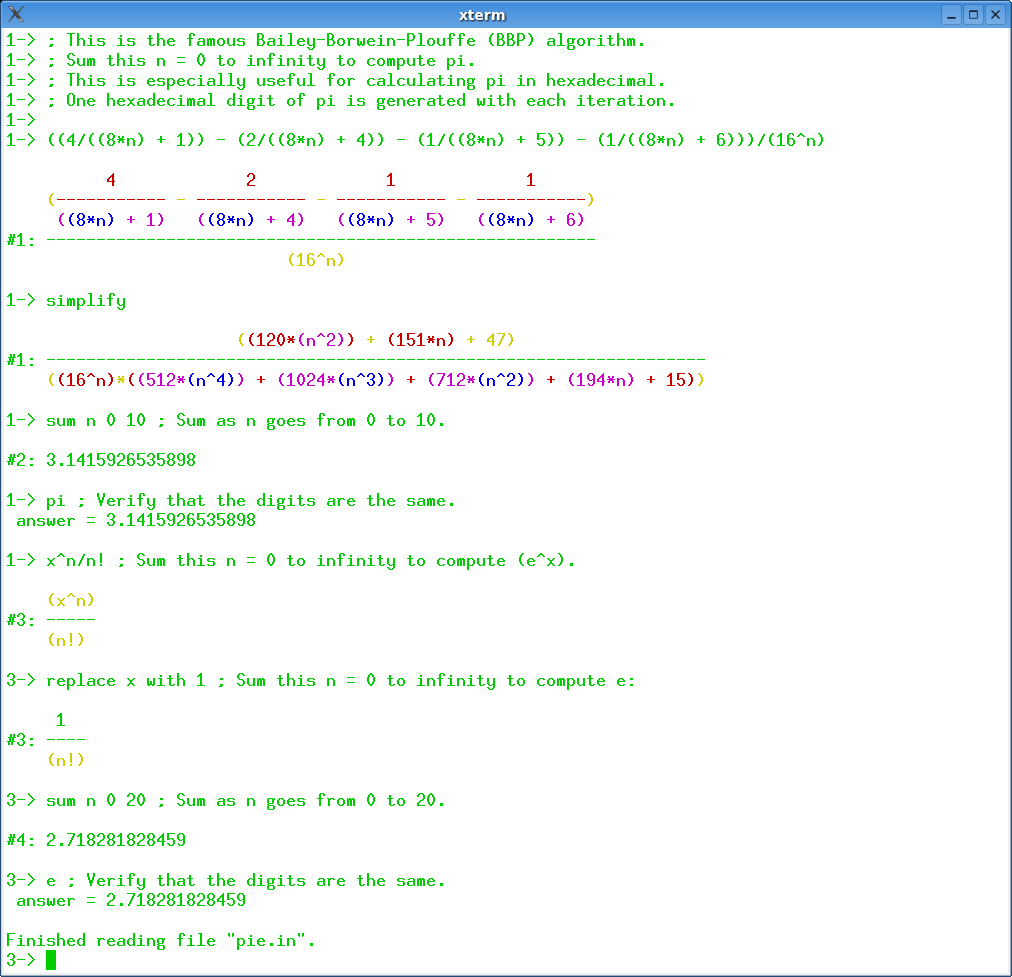## 9 Search Results

### Fermat

Fermat is a super calculator - computer algebra system, in which the basic items being computed can be rational numbers, modular numbers, elements of finite fields, multivariable polynomials, multivariable rational functions, or multivariable polynomials modulo other polynomials. Fermat is available for Mac OS, Windows, Unix, and Linux. It is shareware. The basic “ground ring" F is the field of rational numbers. One may choose to work modulo a specified integer n, thereby changing the ground ring F from Q to Z/n. On top of this may be attached any number of unevaluated variables t_1, t_2, .. t_n., thereby creating the polynomial ring F[t_1, t_2, .. t_n] and its quotient field, the rational functions. Further, polynomials p, q, .. can be chosen to mod out with, creating the quotient ring F(t_1, t_2, ..)/[p, q, ...]. It is possible to allow Laurent polynomials. Once the computational ring is established in this way, all computations are of elements of this ring.

### GAP

GAP is a system for computational discrete algebra, with particular emphasis on Computational Group Theory. GAP provides a programming language, a library of thousands of functions implementing algebraic algorithms written in the GAP language as well as large data libraries of algebraic objects. GAP is used in research and teaching for studying groups and their representations, rings, vector spaces, algebras, combinatorial structures, and more. GAP is developed by international cooperation. The system, including source, is distributed freely under the terms of the GNU General Public License. You can study and easily modify or extend GAP for your special use. The current version is GAP 4, the older version GAP 3 is still available.

### LiE

LiE is the name of a software package that enables mathematicians and physicists to perform computations of a Lie group theoretic nature. It focuses on the representation theory of complex semisimple (reductive) Lie groups and algebras, and on the structure of their Weyl groups and root systems. LiE does not compute directly with elements of the Lie groups and algebras themselves; it rather computes with weights, roots, characters and similar objects.

### Mathomatic

Mathomatic is a free, portable, general-purpose CAS (Computer Algebra System) and calculator software that can symbolically solve, simplify, combine, and compare equations, perform complex number and polynomial arithmetic, etc. It does some calculus and is very easy to use.

More information### NTL

NTL is a high-performance, portable C++ library providing data structures and algorithms for manipulating signed, arbitrary length integers, and for vectors, matrices, and polynomials over the integers and over finite fields.

### RCWA

RCWA is a package for the computer algebra system GAP. It provides implementations of algorithms and methods for computing in certain infinite permutation groups. The class of groups which RCWA in principle can deal with includes the finite groups, the free groups of finite rank, the free products of finitely many finite groups, certain infinite simple groups, certain divisible torsion groups and groups of many further types. It is closed under taking direct products and under taking wreath products with finite groups and with the infinite cyclic group (Z,+).

### SOFA

SOFA - Statistics Open For All The user-friendly, open-source statistics, analysis, and reporting package.